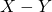# Topics for A-levels H2 MathematicsThe following are a list of A-levels topics in H2 Mathematics. I’ve added what I feel is the hardest part of each topic amongst students.

1. Functions: Finding the range of a composite
2. Curve Sketching: Sketching curves with unknowns and solving conics’ roots.
3. Graphing Transformations: Finding the correct transformation the new graph went through from the original.
4. Inequalities: When there are modulus
5. Arithmetic Progression & Geometric Progression: Finding the general formula for those interest rate questions
6. Summation and Series: MI with Trigo
7. Recurrence Relation: Conjecture
8. Differentiation: Parametric equations
9. Maclaurins’ Series: “Hence” parts which require students to either approximate or relate to standard series.
10. Integration: With trigonometry
11. Definite Integral: Identify the region they want and crafting the correct rotation formula
12. Differential Equations: with given substitution. General vs Particular Solution
13. Vectors I: When no numbers are given, and its just all a, b, c.
14. Vectors II: Distance between two planes, geometrical interpretations.
15. Complex Numbers: Locus of half line. Questions combined with vectors.
16. Probability: story questions which students are still unable to identify the clear events
17. Permutation & Combination: Honestly, don’t spend too long on this questions, beyond 15 minutes. There are too many ways you can suffer at the hands of this question. I can’t even permute it.
18. Normal Distribution: Continuity Correction after approximation
19. Binomial & Poisson Distribution: When we need to findfor a passion distribution we know that we cannot take the difference of two poisson distributions.
20. Hypothesis Testing: Wrong alternate hypothesis
21. Correlation & Regression: Choosing the appropriate model
Not readable? Change text.